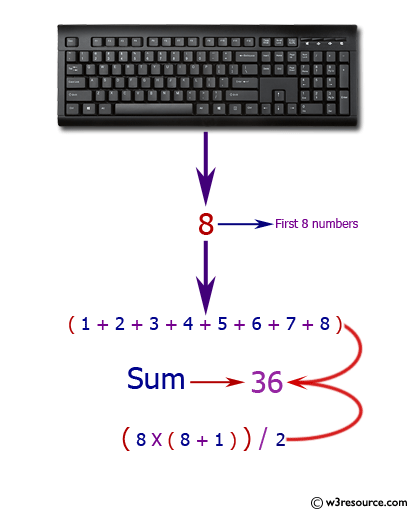﻿ Python: Sum of the first n positive integers - w3resource# Python: Sum of the first n positive integers

## Python Basic: Exercise-58 with Solution

Write a Python program to sum of the first n positive integers.

Pictorial Presentation:Sample Solution-1:

Python Code:

``````n = int(input("Input a number: "))
sum_num = (n * (n + 1)) / 2
print("Sum of the first", n ,"positive integers:", sum_num)
```
```

Sample Output:

```Input a number:  2
Sum of the first 2 positive integers: 3.0
```

## Visualize Python code execution:

The following tool visualize what the computer is doing step-by-step as it executes the said program:

Sample Solution-2:

Python Code:

``````n = int(input("Input an integer: "))
result = sum(range(n+1))
print("Sum of the first", n ,"positive integers:",result)
```
```

Sample Output:

```Input a number:  2
Sum of the first 2 positive integers: 3
```

## Visualize Python code execution:

The following tool visualize what the computer is doing step-by-step as it executes the said program:

Python Code Editor:

Have another way to solve this solution? Contribute your code (and comments) through Disqus.

What is the difficulty level of this exercise?

Test your Python skills with w3resource's quiz

﻿

## Python: Tips of the Day

Unpack variables from iterable:

```# One can unpack all iterables (tuples, list etc)
>>> a, b, c = 1, 2, 3
>>> a, b, c
(1, 2, 3)

>>> a, b, c = [1, 2, 3]
>>> a, b, c
(1, 2, 3)
```